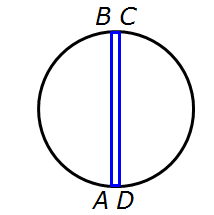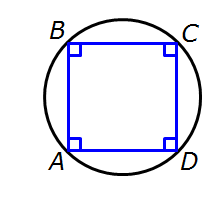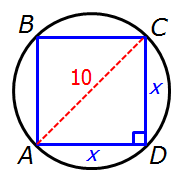It is currently 18 Jun 2019, 08:43GMAT Club Daily Prep

Thank you for using the timer - this advanced tool can estimate your performance and suggest more practice questions. We have subscribed you to Daily Prep Questions via email.

Customized
for You

we will pick new questions that match your level based on your Timer History

Track

every week, we’ll send you an estimated GMAT score based on your performance

Practice
Pays

we will pick new questions that match your level based on your Timer History

Not interested in getting valuable practice questions and articles delivered to your email? No problem, unsubscribe here.The diameter of the circle is 10Question banks Downloads My Bookmarks Reviews Important topics
Author Message
TAGS:
FounderJoined: 18 Apr 2015
Posts: 6898
Followers: 114

Kudos [?]: 1342 , given: 6309

The diameter of the circle is 10 [#permalink]
Expert's post00:00

Question Stats:55% (00:57) correct44% (00:55) wrongbased on 54 sessions
Attachment:#GREpracticequestion The diameter of the circle is 10.jpg [ 7.83 KiB | Viewed 682 times ]

The diameter of the circle is 10

 Quantity A Quantity B The area of the region enclosed by quadrilateral ABCD 40

A)The quantity in Column A is greater.
B)The quantity in Column B is greater.
C)The two quantities are equal.
D)The relationship cannot be determined from the information given.
[Reveal] Spoiler: OA

_________________GRE InstructorJoined: 10 Apr 2015
Posts: 1971
Followers: 60

Kudos [?]: 1798  , given: 9

Re: The diameter of the circle is 10 [#permalink]
3
KUDOS
Expert's post
Carcass wrote:The diameter of the circle is 10

 Quantity A Quantity B The area of the region enclosed by quadrilateral ABCD 40

First of all, it's important not to read too much into the diagram.
All we can glean from the diagram is that we have a quadrilateral that is inscribed in the circle.
That's it!

So, first recognize that the inscribed quadrilateral COULD be a very very very narrow rectangle like this.Notice that this quadrilateral COULD be so thin that its area is very very close to zero.
So, for this particular quadrilateral we get:
Quantity A: a very very small area that's close to zero
Quantity B: 40
In this case, Quantity B is greater.

Alternatively, we COULD make the quadrilateral quite large.
In fact, we could make it a SQUARE.So, if the inscribed quadrilateral is a square, what is its area?
To find out, let's draw a diagonal.One of our circle properties tells us that this diagonal must be the diameter of the circle, which we know is 10
To find the area of the square, we need to know the length of each side.
So, let's let x = the length of each side.

Since ACD is a RIGHT TRIANGLE, we can apply the Pythagorean Theorem to get: x² + x² = 10²
Simplify to get 2x² = 100
Divide both sides by 2 to get: x² = 50

This means the area of the square = 50
We know this because the area of the square = (x)(x) = x², and we just learned that x² = 50

So, for this particular quadrilateral we get:
Quantity A: 50
Quantity B: 40
In this case, Quantity A is greater.

RELATED VIDEO

_________________

Brent Hanneson – Creator of greenlighttestprep.comInternJoined: 12 Jul 2017
Posts: 9
Followers: 0

Kudos [?]: 4 , given: 2

Re: The diameter of the circle is 10 [#permalink]
Could you please explain why we need to consider that the inscribe quadrilateral has a changing size. I mean that can be consider as a small rectangle or a big square.
Thanks so much.
ManagerJoined: 26 Jun 2017
Posts: 104
Followers: 0

Kudos [?]: 41 , given: 38

Re: The diameter of the circle is 10 [#permalink]
But, if we know that the diameter of the circle is 10, then the area of the circle is 25p, is not it? Therefore, we can assume that anything that is inscribed in the circle is going to be lower then 25p.
_________________

What you think, you become.GRE InstructorJoined: 10 Apr 2015
Posts: 1971
Followers: 60

Kudos [?]: 1798  , given: 9

Re: The diameter of the circle is 10 [#permalink]
1
KUDOS
Expert's post
boxing506 wrote:
But, if we know that the diameter of the circle is 10, then the area of the circle is 25p, is not it? Therefore, we can assume that anything that is inscribed in the circle is going to be lower then 25p.

Be careful.
So, if the radius has length 5, then the area of the circle = (pi)(5²) = 25pi ≈ 75
So, if the area of the circle is approximately 75, then the area of the quadrilateral must be LESS THAN 75

Since quantity B is 40, this information (area of the quadrilateral must be LESS THAN 75) doesn't help much.
_________________

Brent Hanneson – Creator of greenlighttestprep.comInternJoined: 09 Jun 2018
Posts: 1
Followers: 0

Kudos [?]: 0 , given: 0

Re: The diameter of the circle is 10 [#permalink]
I have the same question: "Could you please explain why we need to consider that the inscribed quadrilateral has a changing size?" Why do we consider if the inscribed quadrilateral is a square?
InternJoined: 13 Oct 2018
Posts: 21
Followers: 0

Kudos [?]: 0 , given: 4

Re: The diameter of the circle is 10 [#permalink]
sevgi87 wrote:
I have the same question: "Could you please explain why we need to consider that the inscribed quadrilateral has a changing size?" Why do we consider if the inscribed quadrilateral is a square?

I feel it's okay even if you don't consider that it has a changing size. Consider this point instead that you know the diameter is 10 but you don't know if the points C,D LIE on the diameter as the centre is not known. If CD lie on the diameter then it could be solved by taking 2 triangles..( Still a confusion would occur as no data given for sides it could be a square with diagonal as 10 or 6-8-10 pythagorean triplet.) Thus it cannot be determined.Supreme Moderator
Joined: 01 Nov 2017
Posts: 370
Followers: 5

Kudos [?]: 111  , given: 4

Re: The diameter of the circle is 10 [#permalink]
1
KUDOS
Expert's post
Carcass wrote:The diameter of the circle is 10

 Quantity A Quantity B The area of the region enclosed by quadrilateral ABCD 40

A)The quantity in Column A is greater.
B)The quantity in Column B is greater.
C)The two quantities are equal.
D)The relationship cannot be determined from the information given.

Rule to remember
the area of a quadrilateral in a given circle is maximum when the quadrilateral is a square

So the max area of quadrilateral will be when it is square and side of this square will be $$\frac{10}{√2}=5√2$$
So area = $$(5√2)^2=25*2=50$$ , this >40
But minimum can be anything even close to 0.. thus <40

So D
_________________

Some useful Theory.
1. Arithmetic and Geometric progressions : https://greprepclub.com/forum/progressions-arithmetic-geometric-and-harmonic-11574.html#p27048
2. Effect of Arithmetic Operations on fraction : https://greprepclub.com/forum/effects-of-arithmetic-operations-on-fractions-11573.html?sid=d570445335a783891cd4d48a17db9825
3. Remainders : https://greprepclub.com/forum/remainders-what-you-should-know-11524.html
4. Number properties : https://greprepclub.com/forum/number-property-all-you-require-11518.html
5. Absolute Modulus and Inequalities : https://greprepclub.com/forum/absolute-modulus-a-better-understanding-11281.htmlRe: The diameter of the circle is 10   [#permalink] 26 Oct 2018, 20:27
Display posts from previous: Sort by

The diameter of the circle is 10Question banks Downloads My Bookmarks Reviews Important topicsPowered by phpBB © phpBB Group Kindly note that the GRE® test is a registered trademark of the Educational Testing Service®, and this site has neither been reviewed nor endorsed by ETS®.